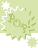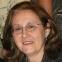Pop-ups: Choose the correct answer from a list of choices.# Squares, Cubes and Fourth Powers of whole numbers

The ^ is used to indicate an exponent.

The ^2 means exponent of 2 or square; ^3 means exponent of 3 or cube the ^4 is exponent of 4.

Remember there is a difference in the evaluating of:
(-3)^2 and -3^2.

(-3)^2 means to square -3 , that is write the -3 down twice and multiply: (-3)(-3) and this is +9.
But the -3^2 means square only the 3. The 3 is written twice not the - .
-3^2= - 3 times 3 = -9.

The phrase "square of -20" is written as (-20)^2 and is +400. The parenthesis are required because the phrase -20^2 means to square only the 20, thus answer is -400.

The exponent tells you how many times to write the BASE. Then you multiply.

3^4 : the 3 is the base and the 4 the exponent.
-3^4: the 3 is the base, write it 4 times.
(-3)^4: the -3 is the base, write it 4 times.

Note the extra negative not inside the parenthesis. -(-4)^2 is not 4^2. It is -1(-4)^2. Which do we do first multiply or exponents? Exponent so we get -1(16) or -16.UsernamePasswordThis activity was created by a Quia Web subscriber. Learn more about QuiaCreate your own activities